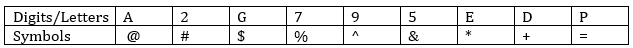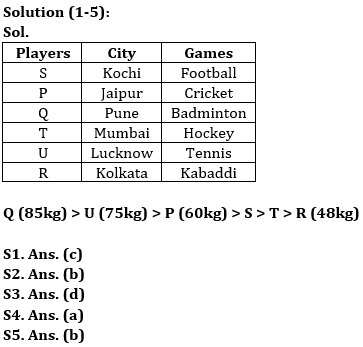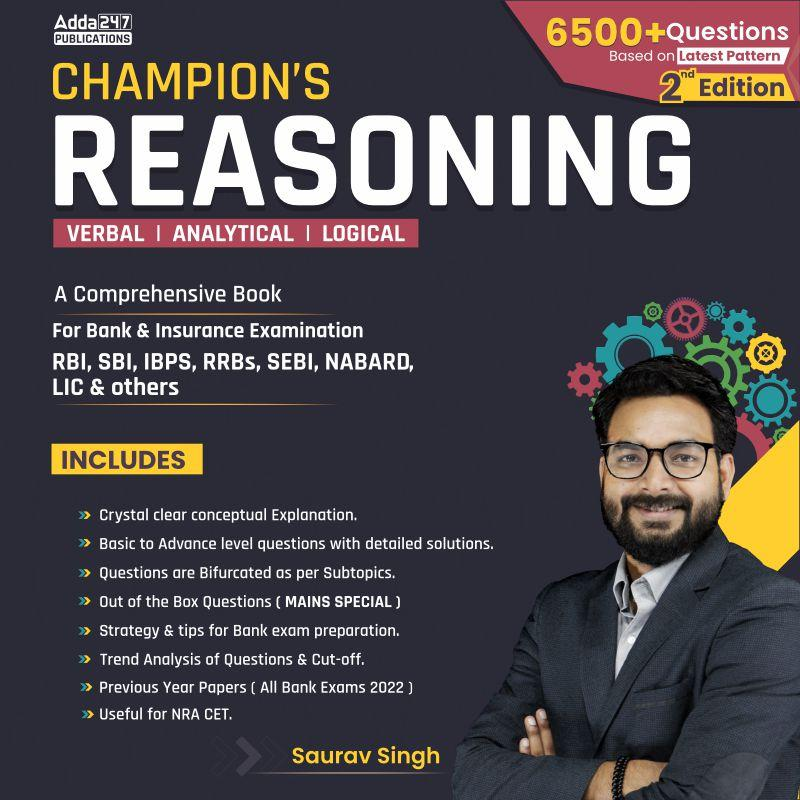Latest Banking jobs   »   IBPS rrb reasoning

# Reasoning Quiz For IBPS RRB Clerk Mains 2023-11th September

Directions (1-5): Read the given information carefully and answer the given questions.
Six players are belonging to different cities. All of them Plays different games. Each player has different weight. S’s weight is more than only 2 persons and he belong to Kochi. The one who belongs to Jaipur has weight which is more than T’s weight but less than U’s weight. The one who likes Cricket, belongs to Jaipur. R doesn’t like Cricket. P’s weight is more than R but less than Q. Q belongs to Pune and T likes Hockey. The one, who is heaviest, has 85kg weight and likes Badminton. T is not the person who has lowest weight. U doesn’t like Badminton. The one who likes Kabaddi doesn’t belong to Kochi and he is not the person who has second highest weight. P’s weight is 60kg. The one who has 75kg weight and likes Tennis and belongs to Lucknow. R doesn’t belong to Mumbai. The one who has lowest weight is 48kg. The one who belongs to Kolkata does not play football.

Q1. Who among the following persons belongs to Kolkata?
(a) S
(b) Q
(c) R
(d) P
(e) None of these

Q2. Who among the following person is 75kg?
(a) Q
(b) U
(c) R
(d) P
(e) None of these

Q3. How many persons are lighter than Q?
(a) None
(b) Two
(c) Four
(d) Five
(e) Three

Q4. The one who plays badminton belongs to which city?
(a) Pune
(b) Jaipur
(c) Mumbai
(d) Lucknow
(e) None of these

Q5. The person who is lightest in the group plays which of the following games?
(a) Cricket
(c) Tennis
(d) Football
(e) None of these

Directions (6-9): In each of the questions given below, a group of digits is given followed by four combinations of symbols numbered (a), (b), (c) and (d). You have to find out which of the four combinations correctly represents the group of digits based on the symbol codes and the conditions given below. If none of the four combinations represents the group of digits correctly, give (e) ‘None of these’ as the answer.Conditions for coding-
(1) If the first element is even number and the last element is vowel then both are to be coded as the code of vowel.
(2) If the first element is odd number and last element is consonant then both are to be coded as the code of the last element.
(3) If the first element is vowel and the last element is consonant then the codes of both elements are to be interchanged.
(4) If the first element is consonant and last element is even number then both are to be coded as the code of the last element.

Q6. DEG7A2
(a) #*\$%@#
(b) +*\$%@+
(c) #*\$@%#
(d) #*\$%@+
(e) None

Q7. 9DE52P
(a) =*+&#=
(b) ^+*&#^
(c) =+*&#=
(d) =+*#&^
(e) None

Q8. 2G795A
(a) @\$^%&@
(b) @\$%^&@
(c) #\$%^&#
(d) @4%^&#
(e) None

Q9. E2PD9G
(a) *#=+^\$
(b)\$ #=+^\$
(c)* #=+^*
(d) \$#=+^*
(e) None

Directions (10): In the given question, a statement is given below followed by two conclusions numbered I and II. You have to assume everything in the statement to be true, then consider the two conclusions together and decide which of them logically follows beyond a reasonable doubt from the information given in the statement.

Q10. Statement: Irregularity is a cause for failure in exams.
Some regular students fail in the examinations.
Conclusions: I. All failed students are regular.
II. All successful students are not regular.
(a) if only conclusion I follows;
(b) if only conclusion II follows;
(c) If either I or II follows;
(d) if neither I nor II follows; and
(e) if both I and II follow.

SolutionsSolutions (6-9):
S6. Ans.(a)
Sol. Here, condition (4) is used
S7. Ans.(c)
Sol. Here, condition (2) is used
S8. Ans.(b)
Sol. Here, condition (1) is used
S9. Ans.(d)
Sol. Here, condition (3) is used

S10. Ans. (d)
Sol. The-given statement clearly implies that all irregular and some regular students fail in the examinations. This, in turn, means that all successful students are regular but not all regular students are successful. So, neither I nor II follows.## FAQs

### When will the IBPS RRB Clerk Mains Exam be conducted?

IBPS RRB Clerk Mains Exam will be conducted on 16 September 2023.

#### Congratulations!Union Budget 2023-24: Free PDF